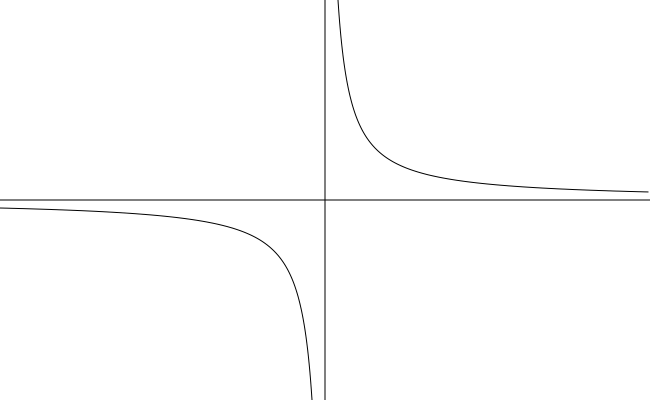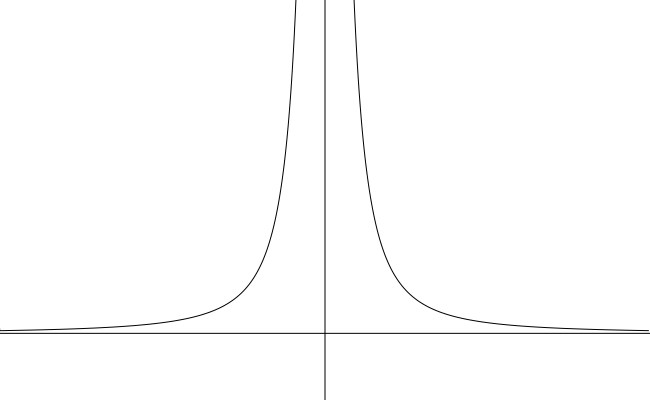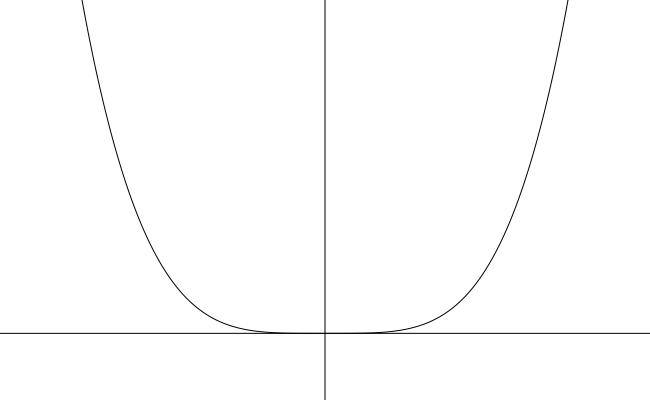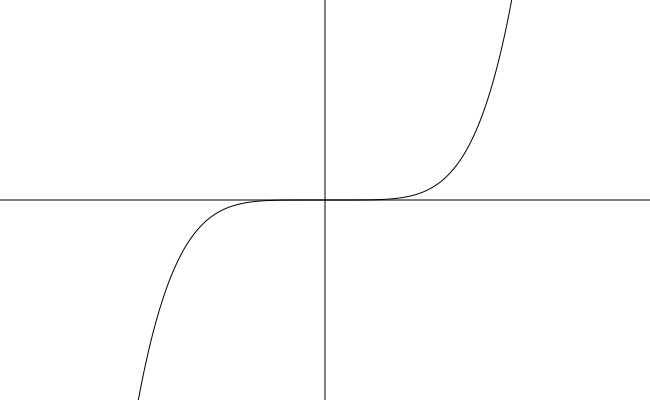# Section 3.2 - Basic Rational & Power Functions and More Transformations

Section Objectives

1. Develop a familiarity with the whole-number power functions and basic rational functions.
2. Apply transformations to basic functions.

### Intro to Polynomials and Rational Functions

An $n$-th-degree polynomial in the variable $x$ is a function of the form

$f(x)=a_nx^n+a_{n-1}x^{n-1}+\cdots+a_1x+a_0$,

where the coefficients $a_n$, $a_{n-1}$, $\dots$, $a_0$ are real or complex numbers with $a_n \ne 0$. We will be almost exclusively interested in polynomials with real coefficients.

Here are some examples of polynomial functions...

• $f(x)=3x^2-6x+1$
• $P(x)=x^8-1$
• $\displaystyle g(x)=\frac{x^3-x^2+x-1}{2}$
• $y=5x-7$
• $y=5$

A rational function is a ratio of two polynomials. That is, a rational function $R$ is a function of the form

$\displaystyle R(x)=\frac{P(x)}{Q(x)},$

where $P$ and $Q$ are polynomials and $Q(x) \ne 0$.

The domain of a rational function is the set of all real numbers for which the denominator is nonzero.

Here are some examples of rational functions...

• $\displaystyle g(x)=\frac{x^3-x^2+x-1}{x^2+1}$
• $\displaystyle y=\frac{5x-7}{x}$
• $y=x^5+2$
• $f(x)=5$

### Reciprocal functions

One of the simplest rational functions is the reciprocal function $\displaystyle y = \frac{1}{x}$.• Domain $=(-\infty,0) \cup (0,\infty)$
• Range $=(-\infty,0) \cup (0,\infty)$
• The reciprocal function is an odd function. Its graph is symmetric about the origin.
• As $x \to \pm \infty$, $\quad y \to 0$. (The $x$-axis is a horizontal asymptote of the graph.)
• As $x \to 0^{+/-}$, $\quad y \to \pm \infty$. (The $y$-axis is a vertical asymptote of the graph.)

Another of the simpler rational functions is the reciprocal square function $\displaystyle y = \frac{1}{x^2}$.• Domain $=(-\infty,0) \cup (0,\infty)$
• Range $=(0,\infty)$
• The reciprocal square function is an even function. Its graph is symmetric about the $y$-axis.
• As $x \to \pm \infty$, $\quad y \to 0$. (The $x$-axis is a horizontal asymptote of the graph.)
• As $x \to 0^{+/-}$, $\quad y \to \infty$. (The $y$-axis is a vertical asymptote of the graph.)

#### Examples

• Sketch the graph of $\displaystyle g(x) = \frac{1}{x-1}+2$. Domain? Range? Asymptotic behavior?

• Determine the horizontal and vertical asymptotes of the graph of $\displaystyle y=\frac{1}{(x+7)^2}-8$.

• Sketch the graph of $\displaystyle f(x) = \frac{-2}{x^2}$.

### Even-number Power Functions: $y=x^n$, $n=2,4,6,\dots$

The graphs of $y=x^2$, $y=x^4$, $y=x^6$, ... are U-shaped curves opening upward with the turning point at the origin.The bigger the exponent, the flatter the curve is on the interval $(-1,1)$ and the steeper the curve is outside that interval.

### Odd-number Power Functions: $y=x^n$, $n=3,5,7,\dots$

The graphs of $y=x^3$, $y=x^5$, $y=x^7$, ... have the following shape. Each has a flat spot at the origin.The bigger the exponent, the flatter the curve is on the interval $(-1,1)$ and the steeper the curve is outside that interval.

#### Examples

• Sketch the graph of $y=(x-2)^4$. Domain? Range? End behavior?

• Sketch the graph of $f(x)=-(x+1)^5$. Domain? Range? End behavior?

• The graph of $y=-x^2-3$ is shifted downward by 7 units. Find an equation for the new graph.

• Let $f(x)=(x+2)^2-4$.
(1) Describe the graph of $f$.
(2) Find the graph's intercepts.
(3) Find the coordinates of the vertex.
(4) Find an equation for the symmetry axis.

• Describe and sketch the graph of $y=-2(x-1)^2+3$.